## Excel VAR.P function

The VAR.P function calculates the variance based on the entire population.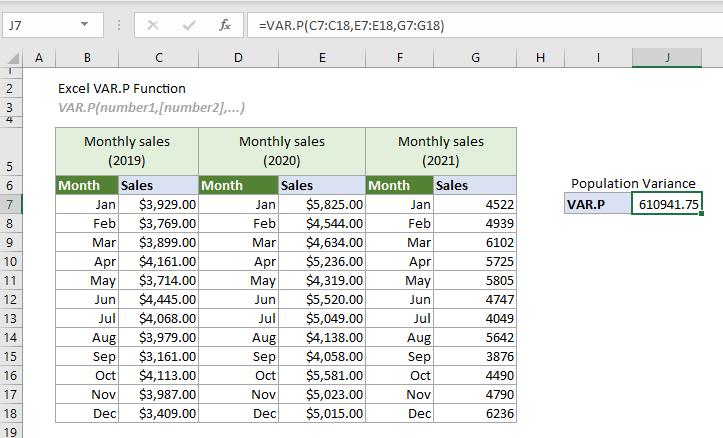#### Excel variance functions

The following table lists all Excel variance functions and their usage notes, which can help you choose the one that best suits your needs.

 Name Data type Text and logicals in references VAR Sample Ignored VAR.S Sample Ignored VARA Sample Evaluated VARP Population Ignore VAR.P Population Ignored VARPA Population Evaluated

#### Syntax

VAR.P(number1, [number2], ...)

#### Arguments

• Number1 (required): The first number or reference used in the calculation.
• Number2, ... (required): The second and more numbers or references used in the calculation.

#### Remarks

1.The VAR.P function was first introduced in Excel 2010 to replace the VARP function. It is available in Excel 2010 and later versions.
2. There can be up to 255 arguments at a time.
3. Arguments can be either of:
-- Numbers;
-- Range names, arrays or cell references that contain numbers.
4. The VAR.P function takes the arguments as the entire population.
Tips: If the data you provided represents a sample of the population, it is recommended to use the VAR.S function.
5. Logical values and text representations of numbers that you directly type in the arguments will be included in the calculation:
-- Logical values: TRUE (1) or FALSE (0);
-- Text representations of numbers: Numbers enclosed in double quotation marks such as “2”.
6. Empty cells, logical values, text, or error values will be ignored in the array or cell references.
Tips: If you want to include logical values, text representations of numbers in the array or cell references as part of the calculation, it is recommended to use the VARPA function.
7. The #VALUE! error occurs if any of the supplied arguments you typed directly in the function are text strings that cannot be interpreted as numbers by Excel.
8. The #DIV/0! error occurs if all supplied arguments are non-numeric.
9. The equation of the VAR.P function is as follows: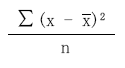Where
-- x represents each value in the sample.
--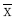is the average of the sample.
-- n is the number of cells in the sample range.

#### Return value

It returns a numeric value.

#### Example

The following table lists the monthly sales for 2019, 2020 and 2021 separately. Assuming that the monthly sales for these three years are the entire population. To calculate the variance based on this entire population, you can apply the VAR.P function as follows to get it done.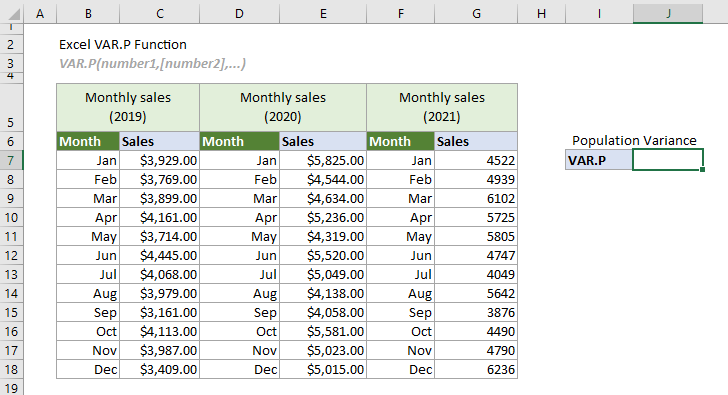Select a cell (says J7 in this case), enter the formula below and press the Enter key to get the result.

=VAR.P(C7:C18,E7:E18,G7:G18)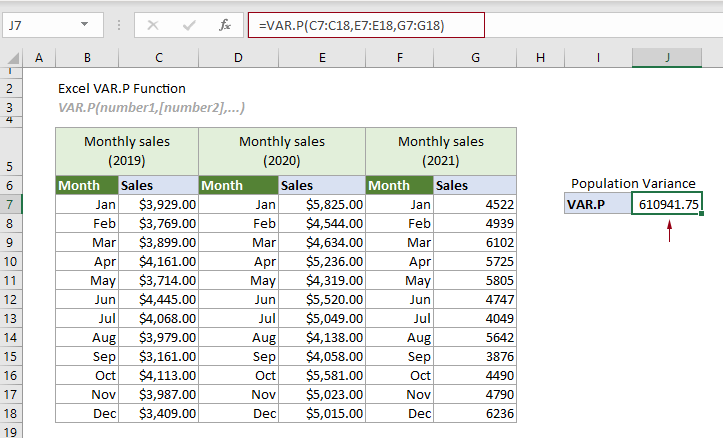Note: Arguments in the formula above are supplied as three cell ranges. However, you can also input the numbers directly in the function as individual numbers or number arrays.

#### Related Functions

Excel VARA function
The VARA function calculates the variance based on a given sample.

Excel VAR function
The VAR function calculates the variance based on a given sample.

Excel VAR.S function
The VAR.S function calculates the variance based on a given sample.

Excel VARP function
The VARP function calculates the variance based on the entire population.

Excel VARPA function
The VARPA function calculates the variance based on the entire population.

### The Best Office Productivity Tools

#### Kutools for Excel - Helps You To Stand Out From Crowd

 Popular Features: Find, Highlight or Identify Duplicates  |  Delete Blank Rows  |  Combine Columns or Cells without Losing Data  |  Round without Formula ... Super VLookup: Multiple Criteria  |  Multiple Value  |  Across Multi-Sheets  |  Fuzzy Lookup... Adv. Drop-down List: Easy Drop Down List  |  Dependent Drop Down List  |  Multi-select Drop Down List... Column Manager: Add a Specific Number of Columns  |  Move Columns  |  Toggle Visibility Status of Hidden Columns  |  Compare Columns to Select Same & Different Cells ... Featured Features: Grid Focus  |  Design View  |  Big Formula Bar  |  Workbook & Sheet Manager | Resource Library (Auto Text)  |  Date Picker  |  Combine Worksheets  |  Encrypt/Decrypt Cells  |  Send Emails by List  |  Super Filter  |  Special Filter (filter bold/italic/strikethrough...) ... Top 15 Toolsets:  12 Text Tools (Add Text, Remove Characters ...)  |  50+ Chart Types (Gantt Chart ...)  |  40+ Practical Formulas (Calculate age based on birthday ...)  |  19 Insertion Tools (Insert QR Code, Insert Picture from Path ...)  |  12 Conversion Tools (Numbers to Words, Currency Conversion ...)  |  7 Merge & Split Tools (Advanced Combine Rows, Split Excel Cells ...)  |  ... and more

Kutools for Excel Boasts Over 300 Features, Ensuring That What You Need is Just A Click Away...#### Office Tab - Enable Tabbed Reading and Editing in Microsoft Office (include Excel)

• One second to switch between dozens of open documents!
• Reduce hundreds of mouse clicks for you every day, say goodbye to mouse hand.
• Increases your productivity by 50% when viewing and editing multiple documents.
• Brings Efficient Tabs to Office (include Excel), Just Like Chrome, Edge and Firefox.